# Week 7 - Chemistry Flashcards

Set Details Share
created 3 years ago by SkyRose
6,215 views
Subjects:
chemistry
Page to share:
Embed this setcancel
COPY
code changes based on your size selection
Size:
X

1

etermine the number of grams of C₄H₁₀ that are required to completely react to produce 8.70mol of CO₂ according to the following combustion reaction: 2C₄H₁₀(g)+13O₂(g)→8CO₂(g)+10H₂O(g)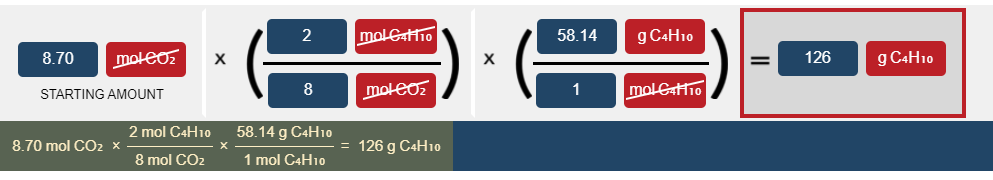The correct answer is 126 g C₄H₁₀.

2

Determine the number of grams of HCl that can react with 0.750g of Al(OH)₃ according to the following reaction

Al(OH)₃(s)+3HCl(aq)→AlCl₃(aq)+3H₂O(aq)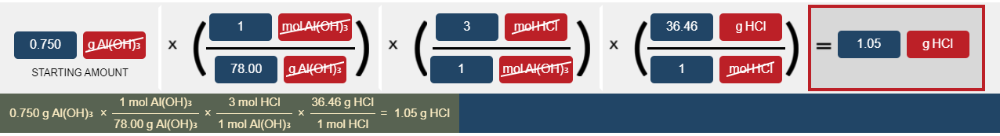The correct answer is 1.05 g HCl.

3

How many grams of oxygen gas are produced when 2.43 × 10⁻⁴g of KClO₃ are completely reacted according to the following chemical equation: 2KClO₃(s)→2KCl(s)+3O₂(g)

9.52 × 10⁻⁵ g O₂.

4

How many grams of nitric acid, HNO₃ ,are required to neutralize (completely react with) 4.30g of Ca(OH)₂according to the acid-base reaction: 2HNO₃(aq)+Ca(OH)₂(aq)→2H₂O(l)+Ca(NO₃)₂(aq)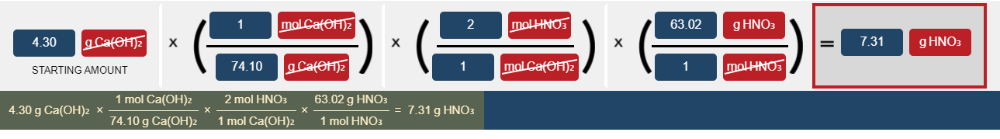The correct answer is 7.31 g HNO₃.

5

How many moles of NH₃ can be produced from 3.76 moles of nitrogen in the following reaction: N₂ (g) + 3 H₂ (g) → 2 NH₃ (g)

7.52 mol

6

How many moles of Al are necessary to form 28.6 g of AlBr₃ from this reaction: 2 Al(s) + 3 Br₂(l) → 2 AlBr₃(s) ?

0.107 mol

7

What is the mass in grams of H₂ that can be formed from 54.6 grams of NH₃ in the following reaction? 2 NH₃(g) → 3 H₂(g) + N₂(g)

9.71 g

8

How many moles of HNO₃ will be produced from the reaction of 45.0 g of NO₂ with excess water in the following chemical reaction? 3 NO₂(g) + H₂O (l) → 2 HNO₃(g) + NO(g)

0.652 mol

9

How many moles of lithium hydroxide would be required to produce 38.5 g of Li₂CO₃ in the following chemical reaction? 2 LiOH(s) + CO₂(g) → Li₂CO₃(s) + H₂O(l)

1.04 mol

10

Milk of magnesia, which is an aqueous suspension of magnesium hydroxide, is used as an antacid in the reaction below. How many molecules of HCl would have to be present to form 75.82 g of MgCl₂? Mg(OH)₂(s) + 2 HCl(aq) → 2 H₂O(l) + MgCl₂(aq)

9.591 × 10²³ molecules

11

Consider the following reaction: C₆H₁₄ + 19/2O₂ → 7H₂O + 6CO₂. Here, C₆H₁₄ is a(n):

A) Reactant

B) Product

C) Hydrocarbon

D) Ion

E) Both (A) and (C)+

E) Both (A) and (C)

12

If you have 1 mol Xe and 1 mol F₂, how many moles of XeF₄ can you create in the following chemical reaction? Xe (g) + 2 F₂ (g) → XeF₄ (g)

A) 2 mol

B) 1 mol

C) 0.5 mol

D) 0.25 mol+

C) 0.5 mol

13

f 2.00 moles of H₂ and 1.55 moles of O₂ react how many moles of H₂O can be produced in the reaction below? 2 H₂(g) + O₂(g) → 2 H₂O(g)

2.00 mol

14

If 0.200 moles of AgNO₃ react with 0.155 moles of H₂SO₄ according to this UNBALANCED equation below, what is the mass in grams of Ag₂SO₄ that could be formed? AgNO₃(aq) + H₂SO₄ (aq) → Ag₂SO₄ (s) + HNO₃ (aq)

31.2 g

15

If 24.7 g of NO and 13.8 g of O₂ are used to form NO₂, how many moles of excess reactant will be left over? 2 NO (g) + O₂ (g) → 2 NO₂ (g)

0.020 mol

16

If 25.0 g of NH₃ and 45.0 g of O₂ react in the following reaction, what is the mass in grams of NO that will be formed?

4 NH₃ (g) + 5 O₂ (g) → 4 NO (g) + 6 H₂O (g)

33.8 g

17

What is the difference between actual yield and theoretical yield?

A) Actual yield has to do only with the reactants of a reaction, and theoretical yield has to do only with the products of a reaction.

B) Actual yield is how much is actually produced in a reaction (it has to be given or measured), and theoretical yield is a calculation that has to be done.

C) Actual yield is how much the reaction produces, and theoretical yield is how much a reaction consumes.

D) Actual yield has to do with how much you can actually get out of a reaction, and theoretical yield is how much you can get out of a reaction if you have maximum starting materials.

B) Actual yield is how much is actually produced in a reaction (it has to be given or measured), and theoretical yield is a calculation that has to be done.

18

You have 2.2 mol Xe and 2.0 mol F₂, but when you carry out the reaction you end up with only 0.25 mol XeF₄. What is the percent yield of this experiment?

Xe(g) + 2 F₂ (g) → XeF₄ (g)

25 %

19

In this reaction: Mg (s) + I₂ (s) → MgI₂ (s) If 2.34 moles of Mg react with 3.56 moles of I₂, and 1.76 moles of MgI₂ form, what is the percent yield?

75.2 %

20

In this reaction: Mg (s) + I₂ (s) → MgI₂ (s), if 10.0 g of Mg reacts with 60.0 g of I₂, and 57.84 g of MgI₂ form, what is the percent yield?

88.1 %

21

What mass (in grams) of aspirin (C₉H₈O₄) is produced from 57.6 g of C₇H₆O₃ assuming 95.0% yield from the reaction below? C₇H₆O₃ (s) + C₄H₆O₃ (s) → C₉H₈O₄ (s) + HC₂H₃O₂ (aq).

71.4 g

22

When copper is heated with an excess of sulfur, copper(I) sulfide is formed. In a given experiment, 1.50 g of copper was heated with excess sulfur to yield 1.76 g copper(I) sulfide. What is the percent yield?

93.7 %

23

Which of the following has the greatest amount of potential energy?

A) a ball at the top of a hill.

B) A ball rolling down a hill.

C) A ball at the bottom of a hill.

D) A ball thrown up and moving into the air.

A) a ball at the top of a hill.

24

Which of the following represents an example of kinetic energy?

A) a ball at the top of a hill.

B) A ball rolling down a hill.

C) A ball at the bottom of a hill.

B) A ball rolling down a hill.

25

Convert 23.4 kJ to calories

5590 cal.

26

Convert 3.20 × 10⁴ J to kilo joules 3.20 × 10⁴J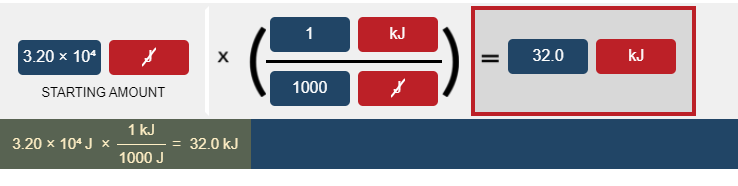The correct answer is 32.0 kJ.

27

The calorie is defined based on the specific heat capacity of what substance?

A) H₂O

B) Al

C) Pb

D) NH₃

E) Hg

A) H₂O

28

How many calories are in 5401 J ? (1 cal = 4.184 J)

1.291 × 10³ cal

29

Which of the following units of energy is defined as the heat it takes to raise the temperature of 1 gram of water by 1° C?

A) kilojoule

B) joule

C) watt

D) kilocalorie

E) calorie

E) calorie

30

The standard enthalpy of formation for one mole of methanol (CH₃OH) is -239 kJ. What is this value in kcal (1 cal = 4.18 J)?

A) Not enough info

B) -57.2 kcal

C) -0.0175 kcal

D) -57,200 kcal

E) -239,000 kcal

B) -57.2 kcal

31

An exothermic reaction causes the surroundings to

A) warm up

B) become acidic

C) condense

D) decrease in temperature

E) release CO₂

A) warm up

32

Which one of the following processes is endothermic?

A) Boiling a liquid.

B) Freezing a solid.

C) Condensation of a gas into a liquid.

D) Condensation of a gas into a solid.

A) Boiling a liquid.

33

Which one of the following processes is exothermic?

A) Making coffee.

B) Baking a cake.

C) Burning wood in a campfire.

D) Photosynthesis.

C) Burning wood in a campfire.

34

Which one of the equations below is an exothermic reaction?

A) CO₂ (g) → C (s) + O₂ (g) ∆H° = 394 kJ/mol

B) CaO (s) + H₂O (l) → Ca(OH)₂ (aq) ∆H° = -64 kJ/mol

C) C (s) + 2 F₂ (g) → CF₄ (g) ∆H° = 141.3 kJ/mol

D) N₂ (g) + O₂ (g) → 2 NO (g) ∆H° = 180.6 kJ/mol

B) CaO (s) + H₂O (l) → Ca(OH)₂ (aq) ∆H° = -64 kJ/mol

35

Equal amounts of heat are added to equal masses of substances A and B at the same temperature, but substance B gets hotter. What is true of their heat capacities (c)?

A) c(A) > c(B)

B) c(B) > c(A)

C) c(a) = c(B)

D) Not enough info

A) c(A) > c(B)

36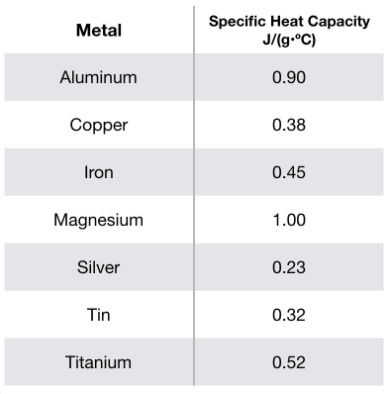What is the identity of a 100. g sample of metal that, upon absorbing 4680 J of heat, increases in temperature by 52.0°C?

A) Mg

B) Al

C) Ti

D) Fe

B) Al

37

How much heat, in kJ, will be absorbed by a 25.0 g piece of aluminum (specific heat = 0.930 J/g･°C) as it changes temperature from 25.0°C to 76.0°C?

A) 5.34 kJ

B) 1190 kJ

C) 1.19 kJ

D) 5340 kJ+

C) 1.19 kJ

38

If 801 J of heat is available, what is the mass in grams of iron (specific heat = 0.450 J/g･°C) that can be heated from 22.5°C to 120.0°C?

18.3 g

39

What is the specific heat of a 75.01 g piece of an unknown metal that exhibits a 45.2°C temperature change upon absorbing 1870 J of heat?

0.552 J/g･°C

40

A 130.3 g piece of copper (specific heat 0.380 J/g･°C) is heated and then placed into 400.0 g of water initially at 20.7°C. The water increases in temperature to 22.2°C. What is the initial temperature (in °C) of the copper? (The specific heat of water is 4.184 J/g･°C).

72.9 °C

41

Which of the following phase changes represents sublimation?

A) gas ⟶ liquid

B) liquid ⟶ gas

C) liquid ⟶ solid

D) solid ⟶ liquid

E) solid ⟶ gas

E) solid ⟶ gas

42

Which of the following phase changes is endothermic?

A) Sublimation

B) Deposition

C) Freezing

D) Condensation

A) Sublimation

43

Which one of the following phase changes would be exothermic?

A) sublimation

B) vaporization

C) condensation

D) melting

C) condensation

44

Which one of the following phase changes would be endothermic?

A) A phase change from a solid to a gas.

B) A phase change from a gas to a liquid.

C) A phase change from a liquid to a solid.

D) None of the above cases are endothermic.

A) A phase change from a solid to a gas.

45

Which one of the following statements would be true about vaporization?

A) It is the phase change from solid to gas.

B) It is an endothermic process.

C) The liquid must release heat as it becomes a gas.

D) None of the above statements are true.

B) It is an endothermic process.